## An archer puts a 0.285 kg arrow in a bow and uses an average force of 182 N to draw the string back 1.32 m. Assume the energy stored in the

Question

An archer puts a 0.285 kg arrow in a bow and uses an average force of 182 N to draw the string back 1.32 m. Assume the energy stored in the bow is transferred to the arrow when it is shot. (a) What is the speed of the arrow as it leaves the bow

in progress 0
4 weeks 2021-08-19T13:49:50+00:00 1 Answers 0 views 0

speed of the arrow as it leaves is 41.05 m/sec

Explanation:

We have given mass m = 0.285 kg

Average force F = 182 N

Distance traveled d = 1.32 m

We know that work done = force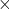distance

Sp work done =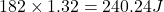Now according to  work energy theorem work done will be equal to kinetic energy

So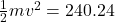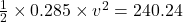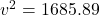v = 41.05 m/sec

So speed of the arrow as it leaves is 41.05 m/sec